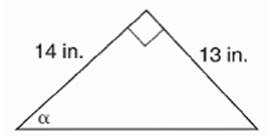Chapter 11.CR, Problem 5CR### Elementary Geometry for College St...

6th Edition
Daniel C. Alexander + 1 other
ISBN: 9781285195698

#### Solutions

Chapter
Section### Elementary Geometry for College St...

6th Edition
Daniel C. Alexander + 1 other
ISBN: 9781285195698
Textbook Problem

# In Exercises 5 to 8, state the ratio needed, and use it to find the measure of the indicated angle to the nearest degree.To determine

To find:

The measure of the angle indicated to the nearest degree.

Explanation

Procedure used:

In any right triangle ABC with mA=θ we have the following ratios.

sinθ=OppositeHypotenuse

Calculation:

Given:

Let us name the above given triangle as ABC

Thus,

mCAB=α,

AB=14 in

### Still sussing out bartleby?

Check out a sample textbook solution.

See a sample solution

#### The Solution to Your Study Problems

Bartleby provides explanations to thousands of textbook problems written by our experts, many with advanced degrees!

Get Started

#### Convert the following percents to decimals. 13.4%

Contemporary Mathematics for Business & Consumers

#### Calculate y'. 46. y=ln|x242x+5|

Single Variable Calculus: Early Transcendentals, Volume I

#### In Exercises 107-120, factor each expression completely. 118. 8a2 2ab 6b2

Applied Calculus for the Managerial, Life, and Social Sciences: A Brief Approach

#### In problems 15-26,evaluate each expression. 15.

Mathematical Applications for the Management, Life, and Social Sciences